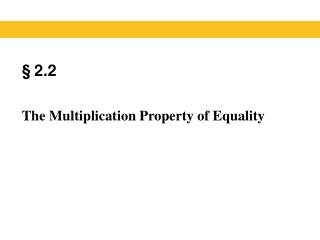Download Presentation§ 2.2

Loading in 2 Seconds...

# § 2.2 - PowerPoint PPT Presentation

§ 2.2. The Multiplication Property of Equality. Properties of Equality. In this section, we introduce an additional property of equality for use in solving equations. This new property is the Multiplication Property of Equality . Blitzer, Introductory Algebra, 5e – Slide # 2 Section 2.2.I am the owner, or an agent authorized to act on behalf of the owner, of the copyrighted work described.
Download Presentation## § 2.2

An Image/Link below is provided (as is) to download presentation

Download Policy: Content on the Website is provided to you AS IS for your information and personal use and may not be sold / licensed / shared on other websites without getting consent from its author.While downloading, if for some reason you are not able to download a presentation, the publisher may have deleted the file from their server.

- - - - - - - - - - - - - - - - - - - - - - - - - - E N D - - - - - - - - - - - - - - - - - - - - - - - - - -
Presentation Transcript
1. §2.2 The Multiplication Property of Equality

2. Properties of Equality In this section, we introduce an additional property of equality for use in solving equations. This new property is the Multiplication Property of Equality. Blitzer, Introductory Algebra, 5e – Slide #2 Section 2.2

3. Multiplicative property of equality EXAMPLE Blitzer, Introductory Algebra, 5e – Slide #3 Section 2.2

4. Dividing both sides by the same nonzero number Since we know that division is the multiplication by the multiplicative inverse, we can divide both sides of an equation by the same number without changing the equation’s solution. Blitzer, Introductory Algebra, 5e – Slide #4 Section 2.2

5. Fractional Coefficients Blitzer, Introductory Algebra, 5e – Slide #5 Section 2.2

6. Using both properties of Equality EXAMPLE Solve: 3x – 10 = - 4x + 18 3x – 10 + 4x = - 4x + 18 + 4xAdd 4x to both sides. 7x – 10 = 18 Simplify. 7x – 10 + 10 = 18 + 10Add 10 to both sides. 7x = 28Simplify. Divide both sides by 7. x = 4 Solve. Blitzer, Introductory Algebra, 5e – Slide #6 Section 2.2

7. Solving Linear Equations Remember the steps for solving linear equations. Blitzer, Introductory Algebra, 5e – Slide #7 Section 2.2

8. Solving Linear Equations EXAMPLE Solve and check: 5 - 3x + 4x = 1 - 7x + 12. SOLUTION 1) Simplify the algebraic expressions on each side. 5 - 3x + 4x = 1 - 7x + 12 Combine like terms: -3x + 4x = x 1 + 12 = 13 5 + x = 13 - 7x Blitzer, Introductory Algebra, 5e – Slide #8 Section 2.2

9. Solving Linear Equations CONTINUED 2) Collect variable terms on one side and constant terms on the other side. 5 + x+ 7x = 13 - 7x+ 7x Add 7x to both sides 5 + 8x = 13 Simplify Subtract 5 from both sides 5 – 5 + 8x = 13 - 5 Simplify 8x = 8 Blitzer, Introductory Algebra, 5e – Slide #9 Section 2.2

10. Solving Linear Equations CONTINUED 3) Isolate the variable and solve. 8x 8 Divide both sides by 8 8 8 x = 1 Simplify Blitzer, Introductory Algebra, 5e – Slide #10 Section 2.2

11. Solving Linear Equations CONTINUED 4) Check the proposed solution in the original equation. 5 - 3x + 4x = 2 - 7x + 6 Original equation ? 5 – 3(1) + 4(1) 1 – 7(1) + 12 = Replace x with 1 ? 5 – 3 + 4 1 – 7 + 12 = Multiply ? 2 + 4 – 6 + 12 = Add or subtract from left to right 6 = 6 Add It checks, and the solution set is {1}. The original sentence is true when x is 1. Blitzer, Introductory Algebra, 5e – Slide #11 Section 2.2